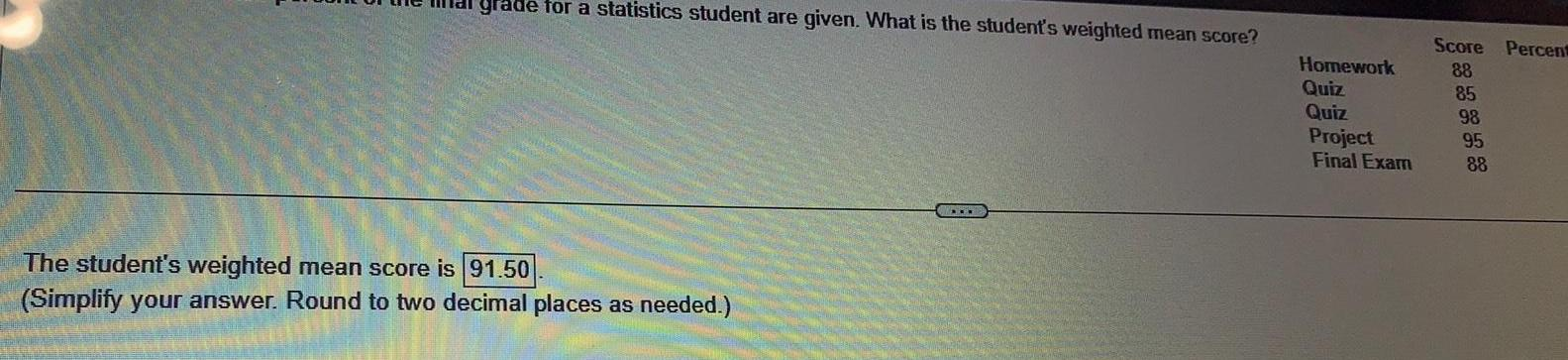Question:

# grade for a statistics student are given What is the student

Last updated: 9/10/2023grade for a statistics student are given What is the student s weighted mean score The student s weighted mean score is 91 50 Simplify your answer Round to two decimal places as needed W Homework Quiz Quiz Project Final Exam Score Percent 88 85 98 95 88